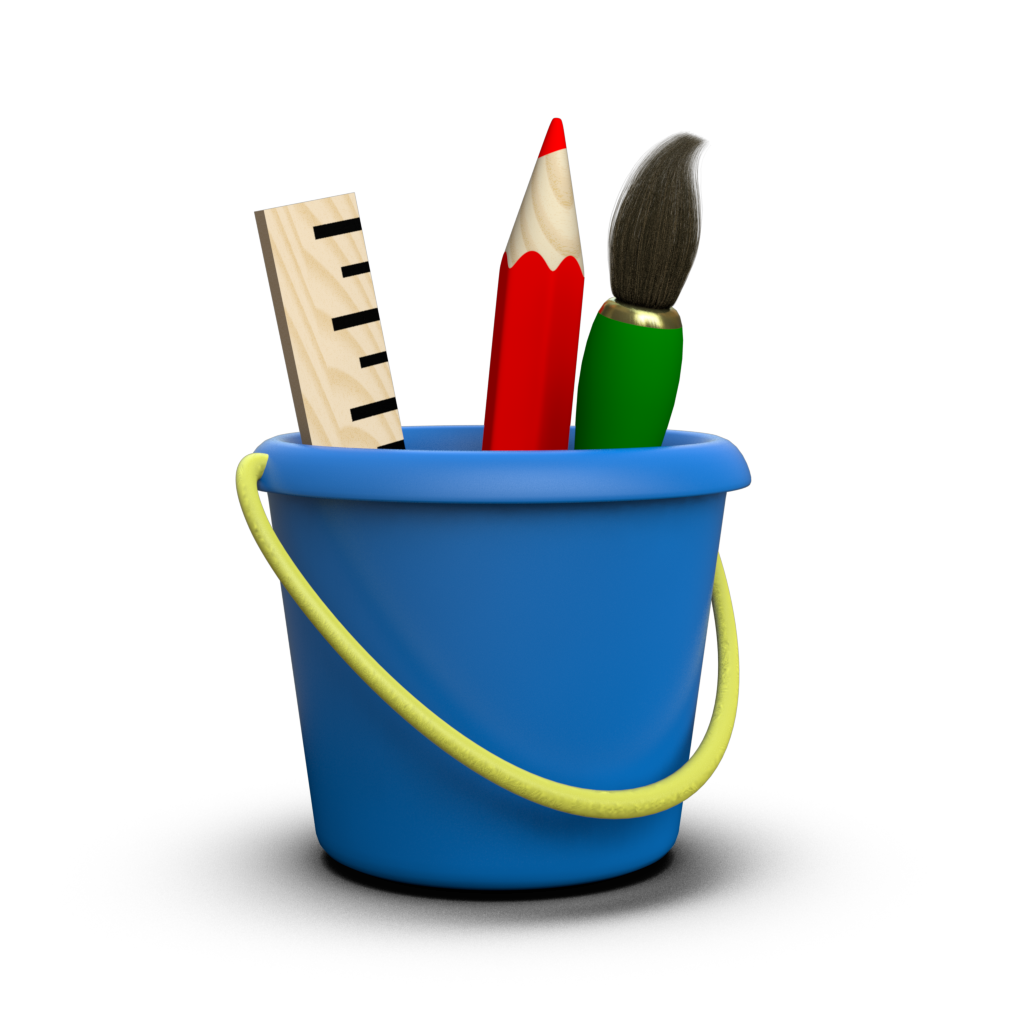# Image Visualization¶

It is often useful to incorporate bitmap images into a visualization. To facilitate this, Toyplot provides toyplot.canvas.Canvas.image() and toyplot.image() functions, which can display image data supplied in a couple of different formats. Note that as a visualization library Toyplot does not provide functionality for reading, writing, or manipulating images - you should do that with libraries such as PIL or scikit-image instead.

For example, you can pass any PIL.Image.Image directly to Toyplot:

:

import PIL.Image
import toyplot

image = PIL.Image.open("../artwork/toyplot.png")
canvas, mark = toyplot.image(image, width=300)


As always, the width parameter in the above example is the width of the Toyplot canvas. The image is then scaled uniformly to fit. Of course, you can use any Canvas Layout method you like to combine images and marks together on the canvas:

:

import numpy

canvas = toyplot.Canvas(width=600, height=300)
mark = canvas.image(image, grid=(1,2,0))
axes = canvas.cartesian(grid=(1,2,1))
axes.plot(numpy.linspace(0, 1) ** 2)
canvas.text(150, 250, "( Toyplot Logo )", style={"font-size":"14px"});